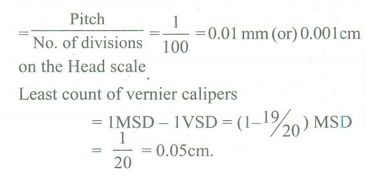Home | | Physics 11th std | Conceptual Questions Answers

## Chapter: 11th Physics : UNIT 1 : Nature of Physical World and Measurement

Physics : Nature of Physical World and Measurement : Book Back Exercise, Example Numerical Question with Answers, Solution : Conceptual Questions Answers

## 1. Why is it convenient to express the distance of stars in terms of light year (or) parsec rather than in km?Answer:The distances of astronomical objects like stars, planets etc from the earth are huge. The distance on the earth are relatively small so it can be measured in km.For Example :The distance to be next nearest big galaxy Andromeda is 21,000,000,000,000,000,000 km. i.e. 21 × 1018 km.This number is so large that it becomes hard to write and to interpret.So astronomical units like light year, parsec A.U are used for large distances.2. Show that a screw gauge of pitch 1 mm and 100 divisions is more precise than a vernier caliper with 20 divisions on the sliding scale.AnswersLeast count of screw gaugeSo screw gauge is more precise than vernier.3. If humans were to settle on other planets which of the fundamental quantities will be in trouble? Why?Answers:Time will be in trouble. Time becomes irrelevant. Because day and year based on spinning and revolution of the planet. So each planet has its own year length.Eg. : Uranus and Neptune move too slow.4. Having all units in atomic standards is more useful. Explain.Answers:All units in atomic standards are more useful because they never change with time.5. Why dimensional methods are applicable only up to three quantities?Answers:If a quantity depends on more than three factors than dimensional formula cannot be derived. Because on equating the powers of M, L & T on either side of the dimensional equation three equations can be obtained, from which only three unknown dimensions can be calculated.

Tags : Nature of Physical World and Measurement | Physics , 11th Physics : UNIT 1 : Nature of Physical World and Measurement
Study Material, Lecturing Notes, Assignment, Reference, Wiki description explanation, brief detail
11th Physics : UNIT 1 : Nature of Physical World and Measurement : Conceptual Questions Answers | Nature of Physical World and Measurement | Physics